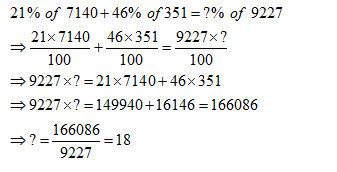• This is an assessment test.
• These tests focus on the basics of Maths and are meant to indicate your preparation level for the subject.
• Kindly take the tests in this series with a pre-defined schedule.

## Basic Maths: Test 57

Congratulations - you have completed Basic Maths: Test 57.

You scored %%SCORE%% out of %%TOTAL%%.

Your performance has been rated as %%RATING%%

 Question 1
$4+44+444+4.444=?$
 A 4936 B 496.444 C 402 D 496
Question 1 Explanation:
$?=4+44+444+4.444=496.444$
 Question 2
21% of 7140 + 46% of 351 = ?% of 9227
 A 56 B 63 C 18 D 69
Question 2 Explanation:Question 3
$11\frac{1}{2}+9\frac{5}{6}-5\frac{2}{3}-2\frac{7}{9}=?$
 A $12\frac{13}{14}$ B $12\frac{8}{9}$ C $12\frac{1}{14}$ D $13\frac{1}{9}$
Question 3 Explanation:
\begin{align} & ?=11\frac{1}{2}+9\frac{5}{6}-5\frac{2}{3}-2\frac{7}{9} \\ & =\left( 11+9-5-2 \right)+\left( \frac{1}{2}+\frac{5}{6}-\frac{2}{3}-\frac{7}{9} \right) \\ & =13+\left( \frac{9+15-12-14}{18} \right) \\ & =13-\frac{2}{18}=13-\frac{1}{9}=12\frac{8}{9} \\ \end{align}
 Question 4
$\left( 0.01\times 0.001\times 0.0001\times {{10}^{10}} \right)$ is equal to
 A 100 B $\frac{1}{10}$ C $\frac{1}{100}$ D 10
Question 4 Explanation:
\begin{align} & =0.01\times 0.001\times 0.0001\times {{10}^{10}} \\ & ={{10}^{-9}}\times {{10}^{10}}=10 \\ \end{align}
 Question 5
$\frac{2.55\times 2.40-2.40\times 2.35}{0.048}$ is equal to:
 A 1 B $\frac{1}{2}$ C $\frac{1}{10}$ D 10
Question 5 Explanation:
\begin{align} & =\frac{2.40\,\left( 2.55-2.35 \right)}{0.048} \\ & =\frac{2.40\times 0.20}{0.048}=10 \\ \end{align}
Once you are finished, click the button below. Any items you have not completed will be marked incorrect.
There are 5 questions to complete.
 ← List →
Shaded items are complete.
 1 2 3 4 5 End